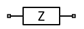# Z (Impedance)

Model complex impedance

•Libraries:
RF Blockset / Circuit Envelope / Elements

## Description

The Impedance block implements the relation, vk(t) = Z(fk)*ik(t), for each simulation frequency, fk, where:

• Z(fk) represents complex-valued impedance at a specified simulation frequency.

• vk(t) represents the voltage across the terminals of the element at time t.

• ik(t) represents the current through the element at time t.

Circuit envelope current and voltage signals comprise in-phase, Ik, and quadrature, Qk, components at each frequency, fk.

Frequency-dependent impedance typically cannot be realized as a physical network such as an RLC chain. You can, however, use the Impedance block to model nonphysical behavior, such as frequency-independent negative capacitance or negative inductance. You can also use this block to specify resonant frequency offsets in filter networks.

## Parameters

expand all

Frequency dependency for the impedance, specified as `Frequency independent` or `Frequency dependent`.

Choose `Frequency independent` to apply the same impedance for all frequencies. Choose ```Frequency dependent``` to apply the impedance as a piecewise linear function.

Data Types: `double`

Impedance value, specified as a scalar complex number in ohms or a vector for complex numbers with each element unit in ohms.

When Impedance type is set to ```Frequency independent```, impedance is a scalar complex number that is applied to all simulation frequencies. When Impedance type is set to ```Frequency dependent```, impedance is a vector of complex numbers, [Z1, Z2, Z3, …]. This vector is linearly interpolated for all simulation frequencies.

Data Types: `double`

Frequency values for frequency dependent impedance, specified as a nonnegative scalar number in Hz or nonnegative vector number with each element unit in Hz.

When Impedance type is set to ```Frequency dependent```, you can specify a vector of nonnegative frequencies, [f1, f2, f3, …]. The pairs, fi, Zi, define a piecewise linear function, Z(f), that is linearly interpolated for the simulation frequencies. For values outside the range, there is constant extrapolation.

Data Types: `double`

## Version History

Introduced in R2010b

expand all